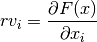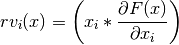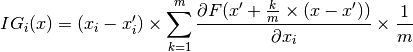# secml.explanation¶

## CExplainer¶

class secml.explanation.c_explainer.CExplainer(clf)[source]

Abstract interface for Explainable ML methods.

Parameters
clfCClassifier

Instance of the classifier to explain.

Attributes
class_type

Defines class type.

clf

Classifier to explain.

logger

Logger for current object.

verbose

Verbosity level of logger output.

Methods

 copy(self) Returns a shallow copy of current class. create([class_item]) This method creates an instance of a class with given type. deepcopy(self) Returns a deep copy of current class. explain(self, x, \*args, \*\*kwargs) Computes the explanation on x. get_class_from_type(class_type) Return the class associated with input type. get_params(self) Returns the dictionary of class parameters. get_state(self) Returns the object state dictionary. get_subclasses() Get all the subclasses of the calling class. list_class_types() This method lists all types of available subclasses of calling one. load(path) Loads object from file. load_state(self, path) Sets the object state from file. save(self, path) Save class object to file. save_state(self, path) Store the object state to file. set(self, param_name, param_value[, copy]) Set a parameter of the class. set_params(self, params_dict[, copy]) Set all parameters passed as a dictionary {key: value}. set_state(self, state_dict[, copy]) Sets the object state using input dictionary. timed([msg]) Timer decorator.
property clf

Classifier to explain.

abstract explain(self, x, *args, **kwargs)[source]

Computes the explanation on x.

class secml.explanation.c_explainer_gradient.CExplainerGradient(clf)[source]

Explanation of predictions via input gradient.

The relevance rv of each feature is given by:• D. Baehrens, T. Schroeter, S. Harmeling, M. Kawanabe, K. Hansen, K.-R.Muller, ” “How to explain individual classification decisions”, in: J. Mach. Learn. Res. 11 (2010) 1803-1831

Parameters
clfCClassifier

Instance of the classifier to explain. Must be differentiable.

Attributes
class_type‘gradient’

Defines class type.

Methods

 copy(self) Returns a shallow copy of current class. create([class_item]) This method creates an instance of a class with given type. deepcopy(self) Returns a deep copy of current class. explain(self, x, y[, return_grad]) Computes the explanation for input sample. get_class_from_type(class_type) Return the class associated with input type. get_params(self) Returns the dictionary of class parameters. get_state(self) Returns the object state dictionary. get_subclasses() Get all the subclasses of the calling class. list_class_types() This method lists all types of available subclasses of calling one. load(path) Loads object from file. load_state(self, path) Sets the object state from file. save(self, path) Save class object to file. save_state(self, path) Store the object state to file. set(self, param_name, param_value[, copy]) Set a parameter of the class. set_params(self, params_dict[, copy]) Set all parameters passed as a dictionary {key: value}. set_state(self, state_dict[, copy]) Sets the object state using input dictionary. timed([msg]) Timer decorator.
explain(self, x, y, return_grad=False)[source]

Computes the explanation for input sample.

Parameters
xCArray

Input sample.

yint

Class wrt compute the classifier gradient.

If True, also return the clf gradient computed on x. Default False.

Returns
relevanceCArray

Relevance vector for input sample.

class secml.explanation.c_explainer_gradient_input.CExplainerGradientInput(clf)[source]

Explanation of predictions via gradient*input vector.

The relevance rv of each features is given by:• A. Shrikumar, P. Greenside, A. Shcherbina, A. Kundaje, “Not just a blackbox: Learning important features through propagating activation differences”, 2016 arXiv:1605.01713.

• M. Melis, D. Maiorca, B. Biggio, G. Giacinto and F. Roli, “Explaining Black-box Android Malware Detection,” 2018 26th European Signal Processing Conference (EUSIPCO), Rome, 2018, pp. 524-528.

Parameters
clfCClassifier

Instance of the classifier to explain. Must be differentiable.

Attributes
class_type‘gradient-input’

Defines class type.

Methods

 copy(self) Returns a shallow copy of current class. create([class_item]) This method creates an instance of a class with given type. deepcopy(self) Returns a deep copy of current class. explain(self, x, y[, return_grad]) Computes the explanation for input sample. get_class_from_type(class_type) Return the class associated with input type. get_params(self) Returns the dictionary of class parameters. get_state(self) Returns the object state dictionary. get_subclasses() Get all the subclasses of the calling class. list_class_types() This method lists all types of available subclasses of calling one. load(path) Loads object from file. load_state(self, path) Sets the object state from file. save(self, path) Save class object to file. save_state(self, path) Store the object state to file. set(self, param_name, param_value[, copy]) Set a parameter of the class. set_params(self, params_dict[, copy]) Set all parameters passed as a dictionary {key: value}. set_state(self, state_dict[, copy]) Sets the object state using input dictionary. timed([msg]) Timer decorator.
explain(self, x, y, return_grad=False)[source]

Computes the explanation for input sample.

Parameters
xCArray

Input sample.

yint

Class wrt compute the classifier gradient.

If True, also return the clf gradient computed on x. Default False.

Returns
relevanceCArray

Relevance vector for input sample.

class secml.explanation.c_explainer_integrated_gradients.CExplainerIntegratedGradients(clf)[source]

Explanation of predictions via integrated gradients.

This implements a method for local explanation of predictions via attribution of relevance to each feature.

The algorithm takes a sample and computes the Riemman approximation of the integral along the linear interpolation with a reference point.

• Sundararajan, Mukund, Ankur Taly, and Qiqi Yan. “Axiomatic Attribution for Deep Networks.” Proceedings of the 34th International Conference on Machine Learning, Volume 70, JMLR. org, 2017, pp. 3319-3328.

So we have for each dimension i of the input sample x:with m the number of steps in the Riemman approximation of the integral.

Parameters
clfCClassifier

Instance of the classifier to explain. Must be differentiable.

Attributes
class_type‘integrated-gradients’

Defines class type.

Methods

 check_attributions(self, x, reference, c, …) Check proposition 1 on attributions. copy(self) Returns a shallow copy of current class. create([class_item]) This method creates an instance of a class with given type. deepcopy(self) Returns a deep copy of current class. explain(self, x, y[, return_grad, reference, m]) Computes the explanation for input sample. get_class_from_type(class_type) Return the class associated with input type. get_params(self) Returns the dictionary of class parameters. get_state(self) Returns the object state dictionary. get_subclasses() Get all the subclasses of the calling class. linearly_interpolate(x[, reference, m]) Computes the linear interpolation between the sample and the reference. list_class_types() This method lists all types of available subclasses of calling one. load(path) Loads object from file. load_state(self, path) Sets the object state from file. save(self, path) Save class object to file. save_state(self, path) Store the object state to file. set(self, param_name, param_value[, copy]) Set a parameter of the class. set_params(self, params_dict[, copy]) Set all parameters passed as a dictionary {key: value}. set_state(self, state_dict[, copy]) Sets the object state using input dictionary. timed([msg]) Timer decorator.
check_attributions(self, x, reference, c, attributions)[source]

Proposition 1:

Attributions should add up to the difference between the score at the input and that at the reference point.

Parameters
xCArray

Input sample.

referenceCArray

The reference sample. Must have the same shape of input sample.

cint

Class wrt the attributions have been computed.

Attributions for sample x to check.

explain(self, x, y, return_grad=<no value>, reference=None, m=50)[source]

Computes the explanation for input sample.

Parameters
xCArray

Input sample.

yint

Class wrt compute the classifier gradient.

referenceCArray or None, optional

The reference sample. Must have the same shape of input sample. If None, a all-zeros sample will be used.

mint, optional

The number of steps for linear interpolation. Default 50.+

Returns

Attributions (weight of each feature) for input sample.

static linearly_interpolate(x, reference=None, m=50)[source]

Computes the linear interpolation between the sample and the reference.

Parameters
xCArray

Input sample.

referenceCArray or None, optional

The reference sample. Must have the same shape of input sample. If None, a all-zeros sample will be used.

mint, optional

The number of steps for linear interpolation. Default 50.

Returns
list

List of CArrays to integrate over.

## CExplainerInfluenceFunctions¶

class secml.explanation.c_explainer_influence_functions.CExplainerInfluenceFunctions(clf, tr_ds, outer_loss_idx='log')[source]

Explanation of predictions via influence functions.

• Koh, Pang Wei, and Percy Liang, “Understanding black-box predictions via influence functions”, in: Proceedings of the 34th International Conference on Machine Learning-Volume 70. JMLR. org, 2017.

Parameters
clfCClassifier

Instance of the classifier to explain. Must provide the hessian.

tr_dsCDataset

Training dataset of the classifier to explain.

Attributes
class_type‘influence-functions’

Defines class type.

Methods

 copy(self) Returns a shallow copy of current class. create([class_item]) This method creates an instance of a class with given type. deepcopy(self) Returns a deep copy of current class. explain(self, x, y[, return_grad]) Compute influence of test sample x against all training samples. get_class_from_type(class_type) Return the class associated with input type. get_params(self) Returns the dictionary of class parameters. get_state(self) Returns the object state dictionary. get_subclasses() Get all the subclasses of the calling class. grad_inner_loss_params(self, x, y) Compute derivative of the inner training loss function for all training points. grad_outer_loss_params(self, x, y) Compute derivate of the outer validation loss at test point(s) x This is typically not regularized (just an empirical loss function) hessian(self, x, y) Compute hessian for the current parameters of the trained clf. list_class_types() This method lists all types of available subclasses of calling one. load(path) Loads object from file. load_state(self, path) Sets the object state from file. save(self, path) Save class object to file. save_state(self, path) Store the object state to file. set(self, param_name, param_value[, copy]) Set a parameter of the class. set_params(self, params_dict[, copy]) Set all parameters passed as a dictionary {key: value}. set_state(self, state_dict[, copy]) Sets the object state using input dictionary. timed([msg]) Timer decorator.
explain(self, x, y, return_grad=False)[source]

Compute influence of test sample x against all training samples.

Parameters
xCArray

Input sample.

yint

Class wrt compute the classifier gradient.

If True, also return the clf gradient computed on x. Default False.

grad_inner_loss_params(self, x, y)[source]

Compute derivative of the inner training loss function for all training points. This is normally a regularized loss.

grad_outer_loss_params(self, x, y)[source]

Compute derivate of the outer validation loss at test point(s) x This is typically not regularized (just an empirical loss function)

hessian(self, x, y)[source]

Compute hessian for the current parameters of the trained clf.

property tr_ds

Training dataset.